# HSSlive: Plus One & Plus Two Notes & Solutions for Kerala State Board

## BSEB Class 12 Physics Wave Optics Textbook Solutions PDF: Download Bihar Board STD 12th Physics Wave Optics Book AnswersBSEB Class 12 Physics Wave Optics Textbook Solutions PDF: Download Bihar Board STD 12th Physics Wave Optics Book Answers

BSEB Class 12th Physics Wave Optics Textbooks Solutions and answers for students are now available in pdf format. Bihar Board Class 12th Physics Wave Optics Book answers and solutions are one of the most important study materials for any student. The Bihar Board Class 12th Physics Wave Optics books are published by the Bihar Board Publishers. These Bihar Board Class 12th Physics Wave Optics textbooks are prepared by a group of expert faculty members. Students can download these BSEB STD 12th Physics Wave Optics book solutions pdf online from this page.

## Bihar Board Class 12th Physics Wave Optics Books Solutions

 Board BSEB Materials Textbook Solutions/Guide Format DOC/PDF Class 12th Subject Physics Wave Optics Chapters All Provider Hsslive

2. Click on the Bihar Board Class 12th Physics Wave Optics Answers.
3. Look for your Bihar Board STD 12th Physics Wave Optics Textbooks PDF.
4. Now download or read the Bihar Board Class 12th Physics Wave Optics Textbook Solutions for PDF Free.

Find below the list of all BSEB Class 12th Physics Wave Optics Textbook Solutions for PDF’s for you to download and prepare for the upcoming exams:

Question 1. Monochromatic light of wavelength 589 nm is incident from air on a water surface. What are the wavelength, frequency and speed of (a) reflected, and (b) refracted light? The Refractive index of water is 1.33. Answer: λ = Wavelength of moncochromatic light = 589 nm, c – speed of light = 3 x 108 ms-1.

(a) For reflected light λ’ = wavelength of reflected light v = frequency of reflected light = ? v = speed of reflected light = ?

(i) As the wavelength of the reflected light remains unchanged, so λ’ = λ = 589 nm = 589 x 10-9 m.

(ii) As the reflection takes place in the same medium, so v = speed of light = C = 3 x 108 ms-1.

(iii) Using relation, C = vλ, we get 𝑣=𝐶𝜆=3×108589×10−9=5.093×1014 Hz

(B) For refracted light—Let λw be the wavelength of light in water vw = frequency of light in water = ? uw = speed of light in water = ?

Using the relation,In moving from one medium to another medium, the frequency of light does not change as the colour of light does not changes, soQuestion 2. What is the shape of the wavefront in each of the following cases: (a) light diverging from a point source. (b) Light emerging out of a convex lens when a point source is placed at its focus. (c) The portion of the wavefront of light from a distant star intercepted by the Earth. Answer: (a) When the light diverging from a point source, then the wavefront is of a converging spherical shape. (b) When the point source is placed on the principal focus of the convex lens, then the rays of light emerging from the lens are parallel to each other, so the wave front will be plane. (c) In this case the shape of the wavefront is almost plane because the source of light i.e., the star is far away from earth, thus a small area on the surface of a large sphere is nearly plane.

Question 3. (a) The refractive index of glass is 1.5. What is the speed of light in glass? (Speed of light in vacuum is 3.0 x 108 m s-1) (b) Is the speed of light in glass independent of the colour of light? If not, which of the two colours red and violet travels slower in a glass prism? Answer: Here, μ = R.I. of glass = 1.5. C = 3.0 x 108 ms-1. ug = speed of light in glass = ? Using the relation, μ = Cv, we get 𝑣g=C𝜇g=3×1081.5=2×108 ms−1

(b) No, the speed of light in glass is not independent of the colour of light. According to Cauchy’s formula, the refractive index depends on the colour i.e., wavelength of light as :as C, a, b are constants.

∴ v α λ2. Thus clearly the speed of light is directly proportional to the square of the wavelength of colour of the light.

Also we know that λv < λr i.e., wavelength of violet colour is lesser than the wavlength of red colour, thus clearly violet colour travels slower in glass than the red component.

Question 4. In a Young’s double-slit experiment, the slits are spearated by 0.28 mm and the screen is placed 1.4 m away. The distance between the central bright fringe and the fourth bright fringe is measured to be 1.2 cm. Determine the wavelength of light used in the experiment. Answer: Here, d = 0.28 mm = 0.28 x 10-3 m. D = Distance between slits and screen = 1.4 m n = order of fringe = 4 y = 1.2 cm = 1.2 x 10-2 m λ = ? For constructive interference, y=n𝜆Dd, we getQuestion 5. In Young’s double-slit experiment using monochromatic light of wavelength X, the intensity of light at a point on the screen where path difference is X, is K units. What is the intensity of light at a point where path difference is 𝜆3? Answer: Here, I = K when path difference = λ I’ = ? When path difference = 𝜆3 We know that the intensity I is given by – I = 2I0 (1 + cos Φ) ……………………………………………………… (1) When Φ = phase difference When path difference is λ, let Φ be the phase difference. ∴ From relation,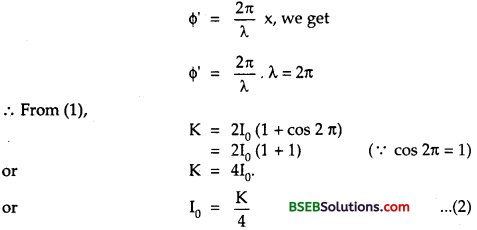Let Φ, be the phase difference for a path difference 𝜆3.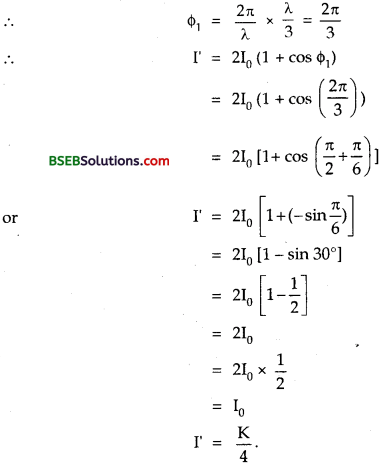Question 6. A beam of light consisting of two wavelengths, 650 nm and 520 nm, is used to obtain interference fringes in a Young’s double-slit experiment. (a) Find the distance of the third bright fringe on the screen from the central maximum for wavelength 650 nm. (b) What is the least distance from the central maximum where the bright fringes due to both the wavelength coincide? Answer: The distance between the slits is 2 mm and the distance between the plane of slits and screen is 120 cm.

Here, λ1 = 650 nm = 650 x 10-9 m = 6500 x 10-10 m. λ2 = 520 nm = 520 x 10-9 m = 5200 x 10-10 m.

(a) n = 3, λ = λ1 = 6500 x 10-10 m. D = 120 cm = 1.20 m, d = 2 mm = 2 x 10-3 m x = distance of third bright fringe from central, maximum = ?

Using the relation,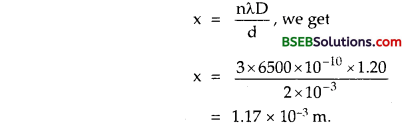(b) Let n be the least number of fringes of λ1 (= 650 nm) which coincide with (n + 1) fringes of λ2 (= 520 nm),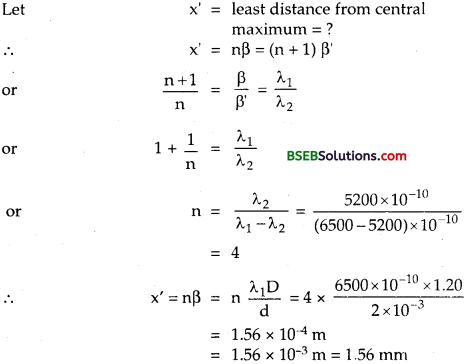Question 7. In a double-slit experiment, the angular width of a fringe is found to be 0.2° on a screen placed 1 m away. The wavelength of light used is 600 nm. What will be the angular width of the fringe if the entire experimental apparatus is immersed in water? Take refractive index of water to be 43. Answer: Here, X = 600 nm = 600 x 10-9 m = 6000 x 10-10 m. θ = angular separation = 0,2′ D = distance of the screen = 1 m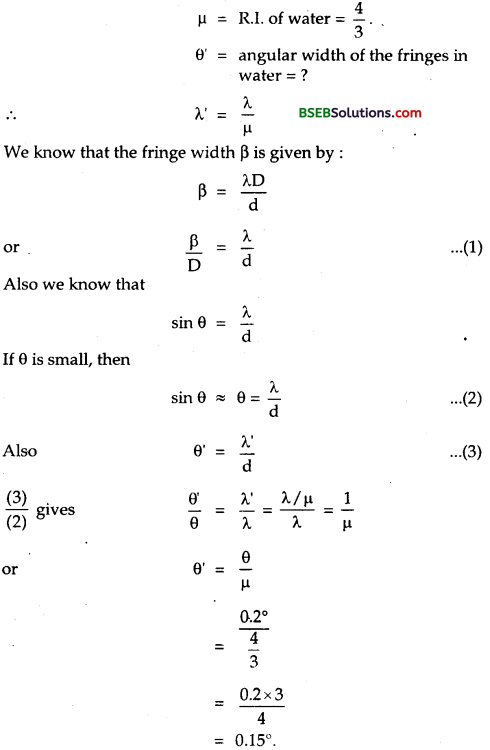Question 8. What is the Brewster angle for air to glass transition? (Refractive index of glass = 1.5). Answer: Here, μg = R.I of glass = 1.5. Let p Brewster angle = ? Also we know that for air to glass transition, μ = tan p ∴ tan p = 1.5 or p = tan-1 1.5 or p = 56.3°.

Question 10.9. Light of wavelength 5000 A falls on a plane reflecting surface. What are the wavelength and frequency of the reflected light? For what angle of incidence is the reflected ray normal to the incident ray? Answer: Here, λ = wavelength of light = 5000 A° = 5000 x 10-10 m. Let λ’, V be the wavelength and frequency of the reflected light. c = Velocity of light in vacuum = 3 x 108 ms-1. If v be the frequency of the incident light, then 𝑣=𝑐𝜆=3×1085000×10−10=6×10710−7 = 6 x 1014 Hz. The wavelength, p and speed of the reflected light remains same, so it frequency (v’) also remains same in all media.Here XY is a plane reflecting surface. OA and AB are the incident and reflected ray. i = angle of incidence r = angle of reflection As AB ⊥ OA, ∴ i + r = 90° Also, according to laws of reflection, r = i ∴ i + i = 90° or 2 i = 90° ∴ 𝑖=902=45∘

Question 10. Estimate the distance for which ray optics is good approximation for an aperture of 4 mm and wavelength 400 nm. Answer: Here, λ = wavelength of light = 400 nm = 400 x 10-9 m a = sizeofaparture=4mm = 4 x 10-3 m Zf = Fresnel’s distance = distance for which the ray optics is good approximation =?

Using the relation, Zf=a2𝜆, we get 𝑍𝑓=(4×10−3)2400×10−9 m=40 m

### Bihar Board Class 12 Physics Chapter 10 Wave Optics Additional Important Questions and Answers

Question 11. The 6563 A Ha line emitted by hydrogen in a star is found to be red-shifted by 15A. Estimate the speed with which the star is receding from the Earth. Answer: Here, λ = Wavelength of Ha lines = 6563 A = 6563 x 10-10 m, C = 3 x 108 ms-1. λ’ – λ = Δλ = red shift = 15 A = 15 x 10-10 m. or λ’ = 1 + Δλ = (6563 + 15) x 10-10 = 6578 x 10-10 m

Red shift takes place when there is recession of the star i.e., when it moves away from earth.

Let v = speed of the star receeding away from earth = ? Δ𝜆=𝑣𝑐𝜆, we get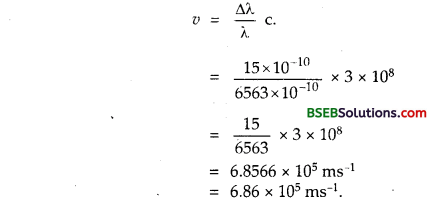Question 12. Explain how Corpuscular theory predicts the speed of light in a medium, say, water, to be greater than the speed of light in a vacuum. Is the prediction confirmed by experimental determination of the speed of light in water? If hot, which alternative picture of light is consistent with the experiment? Answer: According to Newton’s Corpuscular theory, whenever light enters into a denser medium from a rarer medium in the form of particles, a force of attraction comes into play on the particles normal to the surface and hence the component of the velocity normal to the surface of liquid increases whereas the component of the velocity parallel to the surface does not change.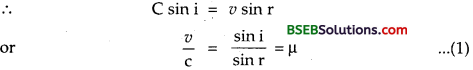where c and v are the speed of light in air and denser medium respectively r are the angles of incidence and angles of refraction respectively, μ = R.I of the denser medium i.e., water (say).

Also we know that μ > 1 ………………………………… (2) From (1) and (2), ∴ 𝑣C > 1 or u > C

i. e., the velocity of light in the denser medium is greater than the velocity of light in the rarer medium.

No, the prediction is not confirmed by the experimental determination of the speed of light in water and thus in actual practice C > v and is in consistent with the experimental data.

Question 13. You have learnt in the text how Huygens’ principle leads to the laws of reflection and refraction. Use the same principle to deduce directly that a point object placed in front of a plane mirror produces a virtual image whose distance from the mirror is equal to the object distance from the mirror. Answer: With the point object at the centre, draw a circle touching the mirror. This is a plane section of the spherical wavefront from the object that has first reached the mirror. Now draw the locations of this same wavefront after a time t in the presence of mirror, and in the absence of mirror. By so doing, we will get two areas symmetrically located on either side of the mirror. Using simple geometry, the centre of the reflected wavefront (the image of the object) is seen to be at the same distance from the mirror as the object.

Question 14. Let us list some of the factors, which could possibly influence the speed of wave propagation : (i) nature of the source. (ii) direction of propagation. (iii) motion of the source and/or observer. (iv) wavelength. (v) intensity of the wave. On which of these factors, if any, does (a) the speed of light in vacuum, (b) the speed of light in a medium (say, glass or water), depend? Answer: (a) The speed of light in vacuum is independent of all these factors as it is a universal constant. (b) Dependence of the speed of light in a medium : (i) The speed of light in a medium is independent of the nature of the source. Wave speed is determined by the properties of the medium of propagation. (ii) The speed of light in a medium is independent of the direction of propagation for isotropic media. (iii) The speed of light in a medium is independent of the motion of the source relative to the medium but depends on the motion of the observer relative to the medium. (iv) The speed of light in a medium depends upon the wavelenth. (v) The speed of light in a medium is independent of the intensity. But this is not valid for high-intensity beams.

Question 15. For sound waves, the Dopier formula for frequency shift differs slightly between the two situations : (i) source at rest; observer moving, and (ii) source moving; observer at rest. The exact Doppler formulas for the case of light waves in vacuum are, however, strictly identical for these situations. Explain why this should be so. Would you expect the formulas to be strictly identical for the two situations in case of light travelling in a medium? Answer: The apparent frequency of lightwave when the source moves towards the stationary observer is the same even when the observer moves towards the stationary. Thus the Doppler’s effect in light is symmetrical. Sound waves require a medium for propagation, thus even though the situations (i) and (ii) may correspond to the same relative motion (between the source and the observer), they are not identical physically since the motion of<the observer), they are not identical physically since the motion of the observer relative to the medium is different in the two situations. Therefore, we cannot expect Doppler formulas for sound to be identical for (i) and (ii). For light waves in a vacuum, there is clearly nothing to distinguish between (iO and ii). Hare only the relative motion between the source and the observer counts and the relative motion between the source and the observed counts and the relativistic Doppler formula is the same for (i) and (ii). For light propagation in a medium, once again like for sound waves, the two situations are not identical and we should expect the Doppler formulas for this case to be different for the two situations (i) and (ii).

Question 16. In a double-slit experiment using light of wavelength 600 nm, the angular width of a fringe formed on a distant screen is 0.1°. What is the spacing between the two slits? Answer: β = Angular fringe width = 0.1° = 0.1 x 𝜋180trad. λ = wavelength of light = 600 nm, = 600 x 10-9 m = 6 x 10-7 m. d = spacing between the slits = ?

∴ Using the relation, 𝛽=𝜆d, we getQuestion 17. Answer the following questions : (a) In a single-slit diffraction experiment, the width of the slit is made double the original width. How does this affect the size and intensity of the central diffraction band? (b) In what way is diffraction from each slit related to the interference pattern in a double-slit experiment? (c) When a tiny circular obstacle is placed in the path of light from a distant source, a bright spot is seen at the centre of the shadow of the obstacle. Explain why? (d) Two students are separated by a 7 m partition wall in a room 10 m high, if both light and sound waves can bend around obstacles, how is that the students are unable to see each other even though they can converse easily. (e) Ray optics is based on the assumption that light travels in a straight line. Diffraction effects (observed when light propagates through small apertures/slits or around small obstacles) disprove this assumption. Yet the ray optics assumption is so commonly used in an understanding location and several other properties of images in optical instruments. What is the justification? Answer: (a) We know that the width of the central diffraction maximum is given by : 𝜆𝐷𝑑 where 𝜆𝑑 is the half angular width of central maximum.

When the width (d) of the slit is made double, then the width of the central diffraction maxima (or band) reduces to half. Thus the area of the central diffraction band would become 14 th. So the intensity of central maximum will become four times i.e. intensity increases four fold. (b) The intensity of interference fringes in a double-slit experiment is the resultant of the diffraction pattern of each slit. (c) A bright spot is seen at the centre of the shadow of the obstacle because the light waves are diffracted from the edges of the circular obstacle. The diffracted waves interfere constructively and give rise to the bright spot. (d) The diffraction is observed by a large-angle when the wavelength of the wave is of the order of the size of the obstacle or aperture. If the size of the obstacle is too much large compared to the wave length, the diffraction is by a small angle. The wavelength of sound wave (= 0,33 m) is larger than the light wave (= 10-7 m) and is also comparable to height of wall or partition, so diffraction of sound waves takes place and hence the students can have conversation with each other easily.

On the other hand, the wavelength of light is very small as compared to the obstacle i.e,, 7m high wall, so the diffraction (bending) of light waves does not take place. Hence seeing one another is not possible.

(e) The justification is based on what is explained in Question 22. (d) i.e., the diffraction of waves by the apertures or obstacles by larger angle takes place when the size of the obstacle is comparable to the wavelength of the wave. The typical sizes of apertures involved in ordinary optical instruments are much larger than the wavelength of light.

Question 18. Two towers on top of top hills are 40 km apart. The line joining them passes 50 m above a hill halfway between the towers. What is the longest wavelenth of radio waves, which can be sent between the towers without appreciable diffraction effects? Answer: Let A1 and A2 be the tops of the two hills. Let O be the another hill half way between the two towers at A1 and A2. s.t.; d = OC = 50 m.∴ From (1) and (2), we getAliter-longest wavelength without diffraction effects is given by:Question 19. A parallel beam of light of wavelength 500 nm falls on a narrow slit and the resulting diffraction pattern is observed on a screen 1 m away. It is observed that the first minimum is at a distance of 2.5 mm from the centre of the screen. Find the width of the slit. Answer: Here, λ = 500 nm = 500 x 10-9 m D = 1m x = distance of first minimum on either side = 2.5 mm x = 2.52 = 1.25 mm = 125 x 10-3 m. = 2.5 x 10-3 m n = 1 d = slit width = ? Using the relation, d sin θ = n λ or d. X/D = 1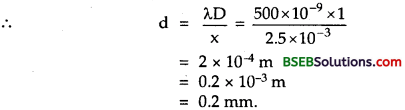Question 20. Answer the following questions : (a) When a low flying aircraft overhead, we sometimes notice a slight shaking of the picture on our TV screen. Suggest a possible explanation.

(b) As you have learnt in the text, the principle of linear superposition of wave displacement is basic to understanding intensity distributions in diffraction and interference patterns. What is the justification of this principle? Answer: (a) When a low flying aircraft passes overhead, it reflects the T.V. signal. The slight shaking on the T.V. screen may be due to the interference taking place between the direct signal received by the internal and the signal reflected by the metallic body of the aircraft.

(b) Superposition principle follows from the linear nature of the differential equation governing the wave motion. The linear combination of wave equations is also a wave equation. If y1 and y2 are the solutions of the wave equation, any linear combination of y1 and y2 is also the solution of the wave equation. This is the very basis of the superposition principle.

Question 21. In deriving the single slit diffraction pattern, it was stated that the intensity is zero at angles of nλ/a. Justify this by suitably dividing the slit to bring out the cancellation. Answer: Divide the single slit into n smaller slits of width ‘a’ given by a’ = 𝑎𝑛, where a is the width of the single slit, n

∴ The angle of diffraction 0 is given by 𝜃=𝑛𝜆𝑎=𝑛𝜆𝑛𝑎′=𝜆𝑎′

Thus each of the smaller slits sends zero intensity in the direction of 0. Hence for the whole single slit, the intensity at angle n𝜆a due to the combination would be zero.

Question 1. Define sustained interference. Answer: It is defined as the interference pattern in which the position of maximum intensity (i.e., constructive interference) and the destructive interference i.e., the position of minimum intensity remain fixed at all times on the screen.

Question 2. Define secondary wavelets. What is their importance? Answer: Every point on the given wave front acts as a fresh source of new disturbance called secondary waveletes.

A surface touching the secondary wavelets in the forward direction at any instant gives the new wavefront at that instant which is called secondary wavefront.

Question 3. Name the phenomenon which establishes the wave nature of light? Answer: Interference, Diffraction and Polarisation are the phenomena which establish the wavenature of light.

Question 4. Define monochromatic source of light. Answer: It is defined as that source of light which produces light of only single wavelength or frequency.

Question 5. Define interference fringes? Answer: They are defined as the alternate equally spread dark and bright bands obtained in the interference patterns.

Question 6. Define coherent sources of light. Answer: They are defined as the sources of light which emit continuous light of same wavelength, same frequency and in the same phase or with a constant phase difference.

Question 7. Define diffraction of light. Answer: It is defined as the phenomenon of bending of light aound the comers of an obstacle or aperture placed in the path of light and spreading into its geometrical shadow.

Question 8. What is the basic difference between the interference and the diffraction? Answer: Diffraction occurs due to the mutual interference of secondary wavelets starting from the different parts i.e., portions of the same wavefront while interference occurs due to the super position of light waves from two coherent sources.

Question 9. Define interference of light. Answer: It is defined as the phenomenon of redistribution of light energy in a medium due to the superposition of light waves from two coherent sources.

Question 10. Why no interference pattern is detected when two coherent sources are infinitely close to each other? Answer: We know that 𝛽∝1 d where d is the spacing between two coherent sources, β = fringe width. Thus as ‘d’ decreases, β increases and when d becomes very small, β becomes very large to the extent that a single fringe may occupy the screen. So the interference pattern may not be detected.

Question 11. Why no interference pattern is detected when two coherent sources are placed far apart? Answer: As 𝛽∝1 d so when d is so large then the fringe width may be reduced beyond the visible region and hence the pattern will not be seen.

Question 12. What is the cause of the luminous border surrounding the profile of a mountain just before the sunrise or sunset? Answer: It is due to the diffraction of light.

Question 13. Can our eyes distinguish between polarised and unpolarised light? Answer: No, our eyes cannot distinguish between polarised anc unpolarised light.

Question 14. What happens to the intensity of light when it is polarised? Why? Answer: The intensity of the polarised light is lesser than that of the intensity of unpolarised light.

Due to polarisation, the vibrations of the electric vector are restricted only in one plane and are cut off in the other planes.

Question 15. Why are short waves used in long-distance broadcasts? Answer: Short waves are used in long-distance broadcasts because they are diffracted less and hence can be transmitted as a beam.

Question 16. Define Fresnel distance. Answer: It is defined as the distance of the screen from the slit when the spreading of light due to diffraction is equal to the size of the slit.

Question 17. Define polarisation of light. Answer: It is defined as the light whose vibrations are restricted only in one plane.

Question 18. Define polarising angle for polarization by reflection. Answer: It is defined as the angle of incidence of light at which the reflected light is completely polarised.

Question 19. Write down the expression for the width of central maximum of the diffraction pattern produced by a single slit. How is it related to the width of the slit? Answer: Width of central maximum =2𝜆𝐷𝑑.

Clearly it is inversely proportional to the width of the slit (d).

Question 20. Define Doppler shift. Answer: It is defined as the apparent change in the frequency or wavelength of light due to the relative motion between the source and the observer.

Question 21. Define Doppler’s effect in light. Answer: It states that whenever there is a relative motion between the observer and the source of light, the apparent frequency of light received by the observer is different from the actual frequency of the ight emitted by the source of light.

Question 22. Define red shift. Answer: It is defined as the shifting of radiations from the source of light towards the red end of the spectrum when the source moves away from the stationary observer. The wavelength increases due to red shift.

Question 23. What is the difference between the unpolarised and polarised light? How are they represented? Answer: In unpolarised light, the vibrations of E→ occur symmetrically in all directions in a plane perpendicular to the direction of propagation of light as represented in the figure.In polarised light, the vibrations of E→ occur only in a particular direction in a plane perpendicular to the direction of propagation as shown belowQuestion 24. Why longitudinal waves cannot be polarised? Answer: The direction of vibration of particles of the medium is along the direction of propagation of the wave in longitudinal wave, so the compressions and rarefractions will pass through the slits used for propagation of light waves.

Question 25. Define plane of polarisation. Answer: The plane which is always perpendicular to the plane of vibrations is called plane of polarisation.

Question 26. State Brewster’s law. Answer: It states that when light is incident at a polarising angle, the reflected and refracted rays are always perpendicular to each other.

Question 27. Define optically active substances. Answer: They are defined as the substances which rotate the plane of polarisation of the incident plane polarised light, e.g., sugar solution.

Question 28. Can we produce interference with sound waves, X-rays and radio waves? Why? Answer: Yes, we can produce interference with sound waves, X-rays and radio waves as interference is the characteristics of wave motion.

Question 29. State two uses of Doppler’s effect of light. Answer: (i) It is used to measure temperature of plasma. (ii) Doppler’s effect is used to measure the speed of recession of a star.

Question 30. Define Crossed Polaroids. Answer: The two polaroids whose plane of polaristion are perpendicular to each other are known as Crossed Polaroids.

Question 31. State the conditions of sustained interference. Answer: (i) The light waves should be of same wavelength. (ii) The two sources should emit the light waves continuously (iii) The waves emitted by two sources of light should either be in phase or should have a constant phase difference, (iv) The light waves emitted should be of the same amplitude. (v) The two sources must lie dose to each other. (vi) The two light sources should be very narrow.

Question 32. Define limit of resolution of an optical instrument. Answer: It is defined as the minimum distance by which the two point objects are separated so that their images can be seen as just separated by the optical instrument.

Question 33. Define resolving power of the optical instruments. Answer: It is defined as the reciprocal of the limit of resolution of the optical instrument.

Question 34. How the resolving power of a telescope changes by increasing or decreasing the aparture of the objective. Answer: We know that the resolving power of a telescope is given by. 𝑅.𝑃.=𝐷1.22𝜆

As R. P α D, so by increasing or decreasing D (aperture) of the objective, the resolving power is increased or decreased.

Question 35. Define a ray of light. Answer: The straight line perpendicular to the wavefront is called ray of light.

Question 36. What is the relation of a wavefront with a ray of light? Answer: The rays of light are always normal to the wavefront.

Question 37. On what factors, does the diffraction depends? Answer: It depends upon the following two factors : (i) Size of the aparture. (ii) Wavelength.

Question 38. How sustained interference pattern is obtained in practice? Answer: Sustained interference is obtained in practice by deriving two sources from a single source or by taking another source as a virtual image of the first source of light.

Question 1. Derive the conditions of interference—Maxima and Minima. Answer: Let y1 = sin ωt …(i) and y2 = a sin (ωt + Φ) ………………………………… (ii) be the displacements of the two waves emitted from the two coherent sources S1 and S2 respectively.

If y be the resultant displacement, thenThus for constructive interference to take place, the phase difference should be even multiple of π.

For I be minimum,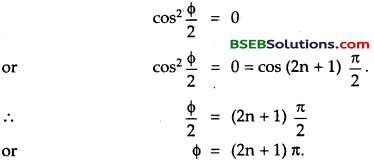Thus for destructive interference to take place, the path difference must be odd multiple of π.

Question 2. Express the conditions of maxima and minima of interference in terms of path difference. Answer: We know that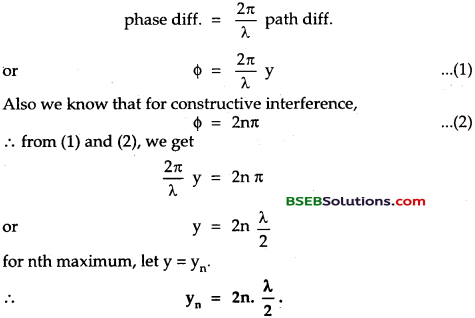i.e., for the constructive interference to take pLace, the path difference must be even multiple of 𝜆2.

For minimum intensity,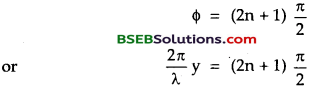Thus for destructive interference to take place, the path difference must be odd multiple of 𝜆2

Question 3. Derive the expression for Fresnel distance. Answer: Let a = slit width λ = wavelength of light used.

The angular position of first secondary minimum called half angular width of the central maximum is given by : 𝜃1=𝜆𝑎 ………………………………… (1)

Let D = distance between the slit and the screen. Then the linear spread of central maximum is given by 𝑦1=𝐷𝜃1=𝐷𝜆𝑎 …………………………. (2)

According to def., When D = Zf y1 = a ∴ a=Zf𝜆a or 𝑍𝑓=𝑎2𝜆

Question 4. Derive the expression for the width of the central maximum of diffraction. ‘ Answer: We know that the distance of nth maximum from centre is given by 𝜃n=𝑦𝑛𝐷 Where D = distance between the slit and the screen. Also we know that 𝜃n=n𝜆d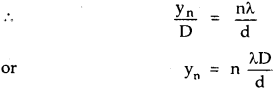Width of secondary maximum is given byWidth of central maximum is given byQuestion 5. Distinguish between interference and diffraction. Answer:

 Interference Diffraction 1. It is due to the superposition of two different wavefronts from two coherent sources, 2. The fringe width is constant. 3. The intensity of all maxima is same. 4. There is a good contrast between the maxima and minima. 1. It is produced due to the superposition of secondary wavelets from the different parts of the same wavefront. 2. The fringes are not of same width. 3. The intensity of different maxima is variable. 4. There is a poor contrast between the maxima and minima.

Question 6. State the importance of Huygen’s principle. What are the two assumptions of this principle? Answer: It is a geometrical construction used to determine the position of the wavefront at any later time from its given position at any time.

Assumptions : (i) Each point on the given wavefront called primary wavefront acts as a source of secondary wavelets sending out the disturbance in all possible directions with the same speed in a similar way as sent by the original source of light,

(ii) The new position of the wavefront at any time is given by the tangent drawn to all the seconary wavelets at that time.

Question 7. What will happen to fringe width when the separation of the slits in Young’s double slit experiment is doubled and the distance between the slits and the screen is halved? Answer: We know that 𝛽=𝜆𝐷𝑑 where d = separation between the slit D = distance of screen from the slit β = fringe width. Now Let d’, D’ and ‘ be the new dimension.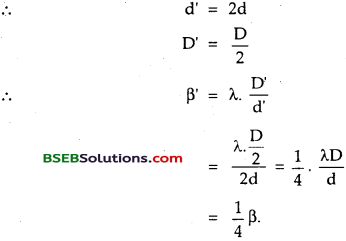The fringe width becomes 14 times the original fringe width.

Question 8. Distinguish between Fresnel and Fraunhofer diffraction. Answer:

 Fresnel Diffraction. Fraunhoffer’s Diffraction 1. Source and screen are placed at finite distances from the aperture. 2. No lens is used. 3. The incident wave front may be spherical or cylindrical. 1. Both the source and the screen are effectively at infinite distance from the slit or aperture. 2. Two convex lenses are used. 3. The incident wavefront is always a plane wave front.

Question 9. In a single slit diffraction pattern, how the angular width of the central bright maxima is changed when : (a) the slit width is reduced. (b) the distance between the slit and the screen is increased. (c) The light of smaller wavelength is used. Answer: We know that the angular width of central maxima is given by: 𝜃=2𝜆d (a) When slit width d decreases, θ will increase. (b) distance between the slit and the screen does not arise in the formula, so the angular width is unaffected. (c) The angular width is directly proportional to the wavelength of light used and hence on using smaller wavelength, angular width will decrease.

Question 10. State and prove Brewster’s law. Answer: It states that when light is incident at a polarising angle at the interface of a refracting medium, the refractive index of the medium is equal to the tangent of the polarising angle i.e.,

μ = tan ip and the reflected light is found to be completely plane polarised.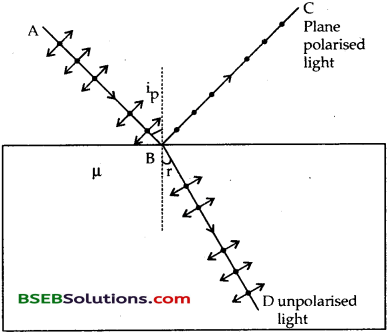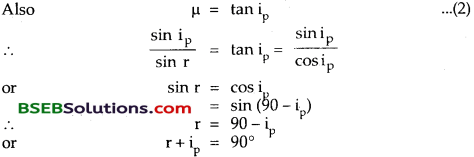i.e., the reflected light is at right angle to the refracted ray when the unpolarised light is incident at the polarising angle.

Question 11. What are the uses of polaroids? Answer: They are used in : (a) Sun glasses – Polaroids are used in sun glasses and are more efficient than those made of coloured glasses. They protect the eyes from the glare.

(b) Wind shields of automobiles—Such wind shields protect the eyes of the driver of the automobile from the dazzling light of the approaching vehicle. (c) In Window panes – They are used in the window panes of aeroplanes and trains to control the light entering through the windows.

(d) In three dimensional motion pictures – The pictures taken by a stereoscopic camera, when seen with the help of polaroid spectacles, create three dimensional effect.

Question 12. Two polaroids are placed 90° to each other and the transmitted intensity is zero. What happens when one more polaroid is placed between these two bisecting the angle between them? What will be the direction of the polarization of the outcoming beam? Answer: Here α = angle between two polaroids = 90° Let I0 be the intensity of light emerging from the first polaroid.

∴ angle made by second polaroid, θ = 45°

If I1 be the intensity of light emerging from the second polaroid, then using Malus law.The third polaroid which is at right angle to the first is now making angle 𝜃′=𝛼2=90∘2=45∘ with the second.

If I2 be the intensity of light coming out of it, theni.e., the intensity is reduced to one fourth of the initial intensity of light i.e., 12 of the intensity emerging from the second polaroid.

Question 1. (a) Derive the expression for the position of interference fringes. (b) Find fringe width. Answer: Let d be the distance between the two coherent sources S1 and S2 respectively. D = distance of the screen from the slits S1 and S2. y = distance of the point P on the screen from the centre O of the screen.

Let x = path difference of the point P on the screen from the centre O of the screen.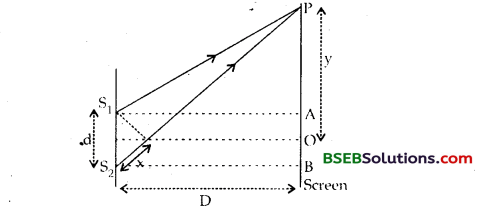Let x = path difference between the light waves reaching at P from S1 and S2.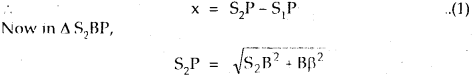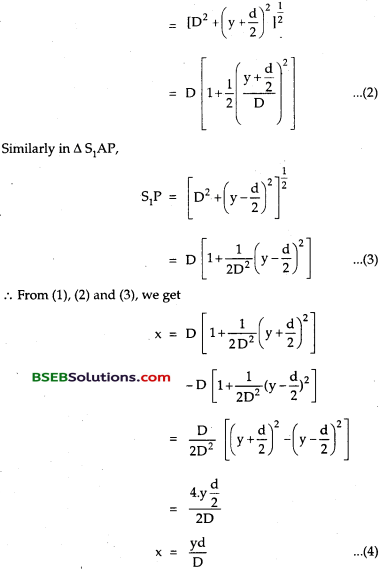Position of maxima and minima on the screen – For bright fringe, x=2n𝜆2Putting n = 0, 1, 2, 3…. for central bright fringe, 1st bright, 2nd bright fringe etc. from point O, we getPutting n = 0, 1, 2, 3…. for central bright fringe, 1st bright, 2nd bright fringe etc. from point O, we get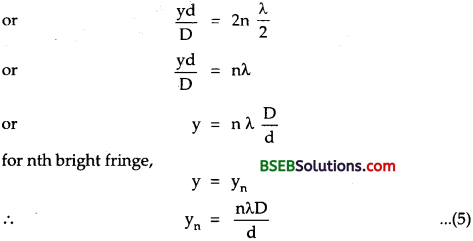for n = O, 1, 2, 3. we get 1st, 2nd, 3rd ….dark fringes as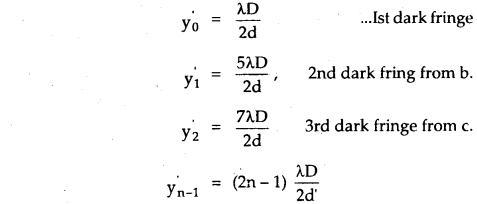(b) Fringe width – For bright fringes,Thus we see that the fringe width of dark fringes is equal to the fringe width of bright fringes.

Numerical Problems

Question 1. Two slits in Young’s interference experiments have widths in the ratio 1 : 9. Deduce the ratio of intensity at the maxima and minima in the interference pattern. Answer: Here, Let W1 and W2 be the widths of two slits. ∴ W1 W2=19 Let a1 and a2 be amplitudes of the waves from there slits.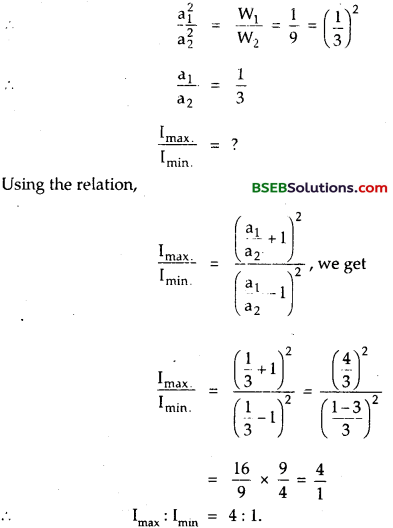Question 2. The ratio of light intensity at the maxima and minima in the interference pattern obtained by Young’s double slit experiment is 9 : 1. Find the ratio of the widths of two slits. Answer: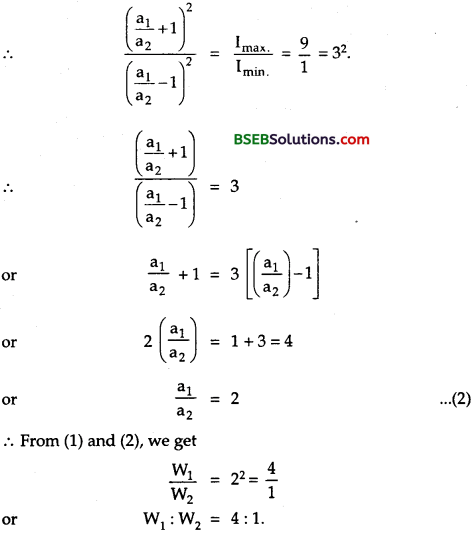Question 3. In Young’s double-slit experiment, while using a source of light of 5000 A wavelength, the fringe width is 0.6 cm. If the distance between the screen and the plane of slit is reduced to half, what should be the wavelength of light to get fringes 0.4 cm wide? Answer: Case I. Here, λ1 = wavelength = 5000 A° β1 = fringe width = 0.6 cm Let D1 = distance between the slits and the screen d= distance between the coherent sources. ∴ Using the relation, 𝛽=𝜆𝐷𝑑, we get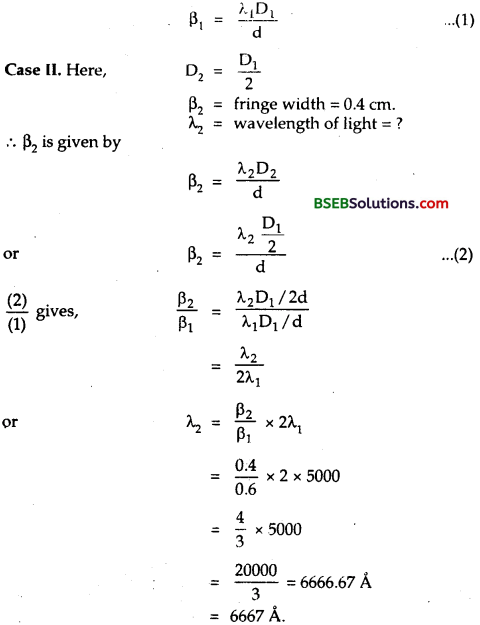Question 4. In Young’s experiment, two coherent sources are placed 0.90 mm apart and the fringes are observed 1 m away. 1f il produces the second dark fringe at a distance of 1 mm from the central fringe, what will be the wavelength of monochromatic light used? Answer: Here, d = distance between two coherent sources = 0.90 mm = 0.9 x 10-3 m.

D = ini for 2nd dark fringe n = 1, yn = y1 = 1mm = 10-3 m λ = ?

For dark fringe, using the relation,Question 5. A slit of width ‘a’ is illuminated by light of wavelength 6000 A°. For what value of ‘a’ will the (a) first maximum fall at an angle of diffraction 30°? (b) First minimum fall at an angle of diffraction of 30°? Answer: Here, λ = 6000 A0 = 6000 x 10-10 m, θ1 = 30°, n = 1. ‘a’ = slit width – ?

(a) For diffraction maximum,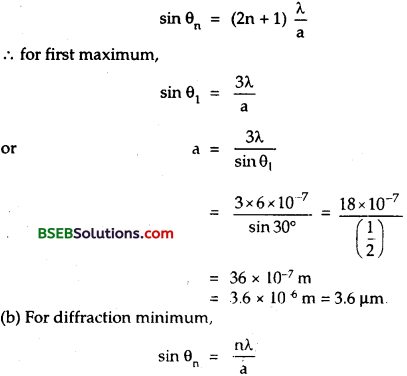Question 6. Light of wavelength 6500 A passes through a slit 0.1 cm wide and forms a diffraction pattern on a screen 1.8 m away. (a) What is the width of the central maximum? (b) Also find the width of the central maximum when the apparatus is immersed in water of refractive index 43. Answer: Here, λ = 6500 A0 = 6500 x 10-10 m = 6.5 x 10-7 m d = slit width = 0.1 cm = 0.1 x 10-2 m = 1 x 10-3 m. D = 1.8 m

(a) Width of the central maximum = ? Width of central maximum = =2𝜆𝐷𝑑=2×6.5×10−7×1.81×10−3 = 2.34 x 10-3 m = 2.34 mm

(b) When the apparatus is immersed in water, then wavelength of light becomes∴ Width of central maximum in this case is given by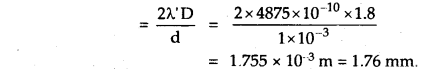Question 7. Light of wavelength 5 x 10-7 m is diffracted by an aparture of width 2 x 10-3 m. For what distance travelled by the diffracted beam does the spreading due to diffraction become greater than the width of the aparture? Answer: Here, λ = wavelength of light = 5 x 10-7 m. d = width of aparture = 2 x 10-3 m. Zf = ? Using the relation,Question 8. In a single slit diffraction experiment, first minimum for red light (660 nm) coincides with first maximum of some other wavelength λ’. Calculate λ. Answer: Here, λ = wavelength = 660 nm = 660 x 10-9 m n = 1 for first diffraction minimum. Using the relation for diffraction minimum,

sin𝜃𝑛=𝑛𝜆𝑑 ∴ sin𝜃1=𝜆𝑑 ……………………………. (1)

For wavelength A’ positions of first maxima is midway between the first and second minima.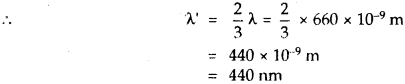Question 9. The spectral line emitted by a star known to have a wavelength of 6500 A°, when observed in the laboratory appears to have a wavelength 62.25 A°. What is the speed of the star in the line of sight relative to the earth? Is the star approaching or receding? Speed of light in air 3 x 108 ms-1. Answer: Here, λ = wavelength = 6500 AC = 6500 x 10-10 m. λ’ = 6525 A = 6525 x 10-10 m. C = 3 x 10-10 ms-1 u = speed of star = ?

Using the relation,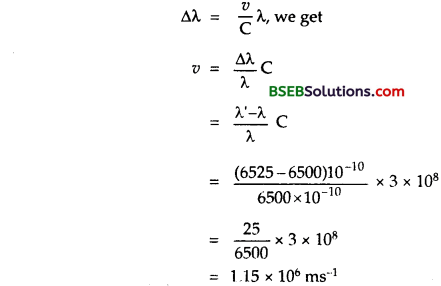As the wavelength is increasing, so the star is receding away from earth.

Question 10. A plane wavefront λ = 6 x 10-7 m falls on a slit 0.4 mm wide. A convex lens of focal length 0.8 m placed behind the slit focuses the light on a screen. What is the linear diameter of: (i) first minimum? (ii) second maximum? Answer: Here, λ = wavelength = 6 x 10-7 m a = slit width = 0.4 mm = 0.4 x 10-3 m f = focal length of the lens = 0.8 m.

(i) Linear diameter of first minimum :(ii) Linear diameter of second maximum :Question 11. Microwave of wavelenth λ = 2.0 cm are incident normally on a slit 5.0 cm wide. Deduce the angular width of the central maximum. Answer: Here, λ = 2.0 cm d = slit width = 5.0 cm 2θ1 = angular width = ? where θ1 is the half angular width of the central maximum and is given by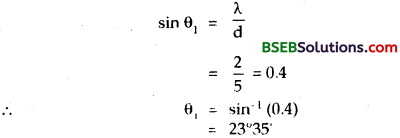∴ The width of the central maximum is given by 2θ1 = 2 x 23°35′ = 47°10’.

Question 12. A double-slit of separation 0.1 cm is illuminated by white light. A coloured interference pattern is formed on a screen 100 cm away. If a pin hole is located on this screen at a distance of 2.0 mm from the central white fringe, what wavelength within the visible spectrum will be absent in the light emitted through the hole? Wavelength in the visible spectra lie between 3500 – 7000 A°? Answer: Here, D = 100 cm yn = 2 mm = 0.2 cm d = 0.1cm The position of nth minima is given by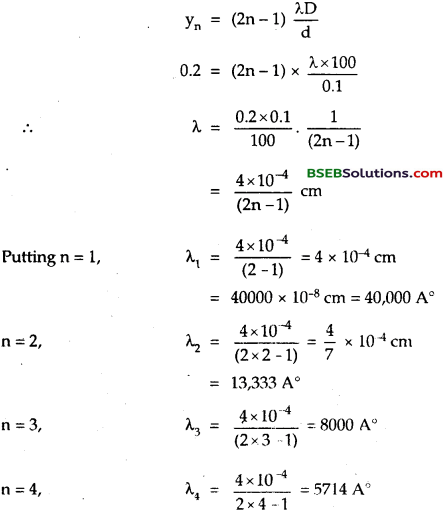We see that for n = 3, λ > 7000 A and for n = 7, λ < 3500 A. So the wavelengths 5714 A, 4444 A and 3636 A will be absent in the visible part of the light transmitted through the pin hole.

Question 13. Critical angle for a certain wavelength of light in glass is 40°. Calculate the polarising angle and the angle of refraction in glass corresponding to it. Answer: Here, C = Critical angle = 40° ip = polarising angle = ? r = angle of refraction = ?

Let μ be the R. I. of glass, ∴ Using the relation,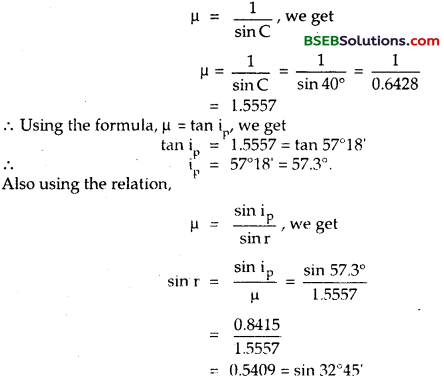Question 14. A parallel beam of monochromatic light of wavelength 450 nm passes through a long slit of width 0.2 mm. Find the angular divergence in which most of the light is diffracted. Answer: Here, λ = wavelength = 450 nm = 450 x 10-9 m d = slit width = 0.2 mm = 0.2 x 10-3 m angular divergence = 2θ = ?

Most of the light is diffracted between the first order minima on either side of the central maximum, for whichQuestion 15. In Young’s experiment, two coherent sources are 1.5 mm apart and the fringes are obtaned at a distance of 2.5 m from them. If the sources produces light of wavelength 589.3 nm, find the number of fringes in the interference pattern which is 4.9 x 10-3 m long. Answer: Here, λ = 589.3 nm = 589.3 x 10-9 m. d = distance between two coherent sources = 1.5 mm = 1.5 x 10-3 m D = 2.5 m x = interference pattern length = 4.9 x 10-3 m Let n = number of interference fringes = ? Let β – fringe width

∴ Using the relation,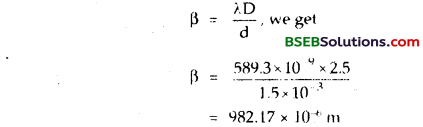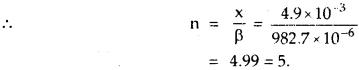Question 16. A double slit arrangement produces interference fringes for sodium light λ = 5890 A0 that are 0.40° apart. What is the angular fringe separation, if the entire arrangement is immersed in water of 𝜇=43? Answer: Here, λ = 5890 A° = 5890 x 10-10 m. θ = angular separation = 0.40° 𝜇=43 θ = angular fringe separation in water = ? We know that the fringe width P is given by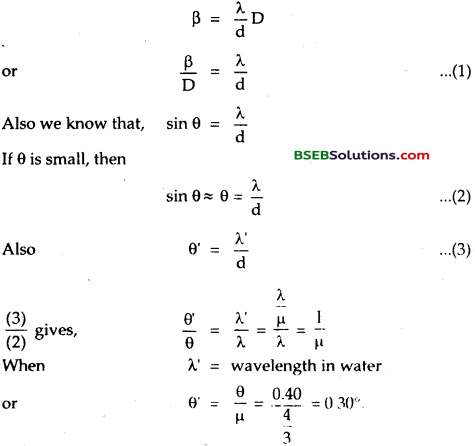Question 17. What is the minimum angular separation between two stars, if a telescope is used to observe them with an objective of aparture 0.2 m? The wavelength of light used is 5900 A. Answer: Here, D = diameter of the objective of telescope = 0.2 m λ = Wavelength of light used 5900 A° = 5900 x 10-10 m

Let dθ = minimum angular separation ‘ between two stars = ? Using the relation,Question 18. (a) Light of wave length 5.4 x 10-5 cm passes through a slit 0.12 cm wide and forms a diffraction pattern on a screen 2.7 m away. What is the width of the central maxima? (b) If the system is immersed in a liquid of refraction index 1.35, what would be the width of the central fringe? Answer: (a) Here, λ = 5.4 x 10-5 cm = 5.4 x 10-7 m d = slit width – 0.12 cm = 12 x 10-4 m D = distance of the screen = 2.7 m β0 = Width of central maxima = ?

Using the relation,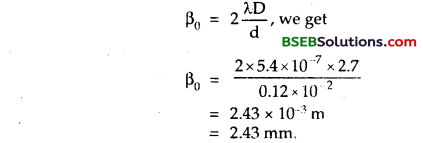(b) When the system is immerged in a liquid, μ of liquid = 1.35

Let β0 = fringe width of the system when immersed in liquid = ?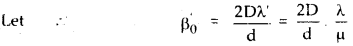Question 19. Light of wavelength 5 x 10-7 m falls on a pair of narrow slits separated by a distance d. The interference pattern on a screen placed 2.0 m away shows that there is darkness at the positions exactly opposite each slit. Calculate the separation’d’ between the slits. Answer: Here, λ = wavelength of light = 5 x 10-7 m d = ?

D = Distance between the slits and the screen = 2m. According to the statement,Question 20. Waves from two different sources overlap near a particular point. The amplitude and the frequency of the two waves are the same. Calculate the ratio of the intensity when the two waves arrive in phase to that when they arrive 90° out of phase. Answer: Let a1 and a2 be the amplitudes of the two waves overlapping each other. Given : a1 = a2 = a (Say)

(i) When they superpose each other in the same phase. Let I1 be the intensity of light in the case so θ = 0.

If A1 be the amplitude in this case, then

Using the relation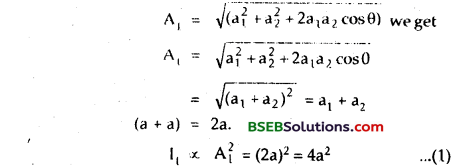Also let I2 and A2 be the intensity of light and amplitude of the resultant wave. When two waves superpose each other 90° out of phase.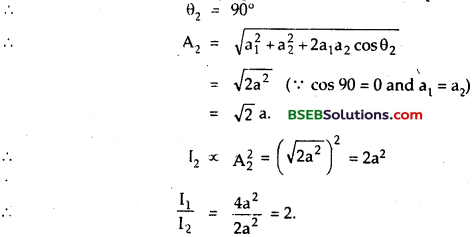Question 21. A rocket is going away from the earth at a speed of 106 m/s. 1f the wavelength of the light wave emitted by it be 5700 A°, what will be its Doppler’s shift? Answer: Here, u = speed of rocket = 106 ms-1 A wavelength of light wave emitted = 5700A° C = 3 x 108 ms-1. Δλ = Doppler shift =?

Using the relation,Fill In The Blanks

Question 1. Width of the central diffraction maximum on immersing the apparatus in a daiserm edium of lefidctiye index g becomes ………………………….. its width in air and wavelength becomes …………………………. . Answer: 1𝜇 times, 1𝜇 times the wavelength

Question 2. The half angular width 0, of the central maximum is given by ………………………… . Answer: sin θ1 = 𝜆𝑑

Question 3. For studying the spectrum of ultra-violet region, the lenses and prisms are made of ………………………………………. . Answer: quartz.

Question 4. Path difference = ………………………………………. x phase difference. Answer: 2𝜋𝜆

Question 5. The path difference between two waves at a pointon the screen for a wavelength λ, is 5𝜆2. The point of the screen is and the order of …………………………… band is ………………………….. . Answer: Minima, dark, third

Question 6. The dark lines in solar spectrum are called …………………………… lines. Answer: Fraunhofer.

Question 7. If the critical angle of a crystal is 45°, the polarising angle is ………………………….. . Answer: tan-1 2‾√

Question 8. The refractive index is equal to the tangent of the angle of polarisation. It is called …………………………… law. Answer: Brewster’s.

Question 9. The wave theory of light in its original form was first postulated by …………………………… Answer: Christian Huygens.

Question 10. A ray of light incident at an angle of incidence i on one surface of a prism of small angle A and emerges normally from the opposite surface. The refractive index of the prism material is p. The angle of incidence i and 0 angle of prism are related as ………………………….. . Answer: i = μA (Hint: δ = (μ – 1)A; For normal emergence, e = o from relation, A + δ = i + e, we get i = A + δ = A + (μ – 1) A = μA)

Question 11. In Young’s double slit experiment, the pringe width is minimum for …………………………… colour. Answer: Violet.

Question 12. In propagation of light waves, the angle between the plane of vibration and the plane of polarization is ………………………….. . Answer: 90°

Question 13. Light waves spreading from two sources produce interference only if they have ………………………….. . Answer: Same frequency and constant phase difference.

Question 14. The refracting angle of a biprism is nearly equal to ………………………….. . Answer: 180°

Question 15. A light of frequency 5 x 1014 Hz, enters a medium of refractive index 1.5. In this medium the velocity of light wave is cm/s and it wavelength is ……………………………. A. Answer: 2 x 1010, 4000

Question 16. Non, reflecting glass owes its property to …………………………… interference. Answer: Destructive.

Question 17. Fringe width of the fringes is …………………………… when the apparatus is immersed in water. Answer: decreased.

Multiple Choice Questions

Question 1. Interference was first observed in the interference chamber, when air was present, now the chamber is evacuated, and if the same light is used then a careful observer will see: (a) no interference (b) interference in which breadth of the fringe will be slightly increased. (c) interference with bright bands. (d) interference with dark bands. Answer: (b) (Hint: μair is slightly more than one, p decreases on evacuation, ∴ X increases and hence the β.)

Question 2. Huygen’s wave theory of light can not explain : (a) Photoelectric effect (b) interference, (c) diffraction (d) polarisation Answer: (a) (Hint: photoelectric effect can be explained on the basis of quantum theory.)

Question 3. Wave optics is valid when characteristic dimensions are : (a) of the order of 1 mm. (b) of the same order as the wavelength of light. (c) much smaller than the wavelength of light (d) much larger than the wave length of light. Answer: (b)

Question 4. Ray optics is valid when the characteristic dimensions are : (a) of the order of 1 mm. (b) of the same order as the wavelength of light. (c) much smaller than the wavelength of light. (d) much larger than the wavelength of light. Answer: (d)

Question 5. The interference pattern is obtained on a screen due to two identical coherent sources of monochromatic light. The intensity of the central bright fringe is 1. When one of the sources is blocked, its intensity becomes IQuestion The intensity in two situations is related as: (a) I = 2 I0 (b) I = I0 (c) I = 4I0 (d) I = 3I0 Answer: (c) (Hint: 1 α (a1 + a2)2 and I0 αa2.4a2)

Question 6. If a source of light is moved away from a stationary observer, then the frequency of light wave appears to change because of: (a) interference (b) diffraction. (c) Doppler’s effect (d) All of these Answer: (c)

Question 7. When seen in green light, the saffron and green portions of our. National Flag will appear. (a) green (b) black (c) black and green respectively. (d) green and yellow respectively. Answer: (c) (Hint: green + saffron = Blackish, green + geen = green.)

Question 8. Light travels through a glass plate of thickness t and having a refractive index μ. If c be the velocity of light in vaccum, the time taken by light to travel this thickness of glass is : (a) tc𝜇 (b) 𝐭𝝁𝑐 (c) t𝜇c (d) t 𝜇c Answer: (a) (Hint: 𝑣=𝑐𝜇,𝑡′=𝑡𝑣=𝑡𝑐𝜇)

Question 9. In Young’s Double Slit experiment using sodium light (λ = 5898 A), 92 fringes are seen. If green colour (546lA) is used, how many fringes will be seen? (a) 62 (b) 99 (c) 67 (d) 85 Answer: (b) (Hint: Using 𝑛1𝑛2=𝜆1𝜆2, ∴ n2=𝜆1𝜆2n1=58985461×92)

Question 10. Young’s experiment established that: (a) light consists of waves. (b) light consists of particles. (c) light consists of both waves and particles. (d) none of these Answer: (a)

Question 11. The condition for observing Fraunhofer diffraction from a single slit is that the light wavefront incident on the slit should be: (a) spherical (b) cylindrical (c) plane (d) none of these Answer: (c)

Question 12. Which of the following establishes the transverse nature of light. (a) polarisation (b) interference (c) Diffraction (d) refraction Answer: (a)

Question 13. Light appears to travel in straight line since : (a) Its wavelength is very large. (b) Its wavelength is very small. (c) It is absorbed by atmosphere. (d) none of these Answer: (b)

Question 14. If n represents the order of a half period zone, the area of this zone is nearly proportional to nm. where m is equal to : (a) 1 (c) 1/2 (d) zero Answer: (d) (Hint: The area of each half period zone is nearly the same, i.e. the area of 1st half period = area of the nth half period zone. ∴ m in n3 must be zero.)

Question 15. Two coherent monochromatic light beams of intensities I and 41 are superimposed. The maximum and minimum possible intensities in the resulting beam are : (a) 91 and I (b) 5 I and I (c) 91 and 31 (d) 5 I and 31 Answer: (a)

Question 16. Two waves, orginating from sources S1 and S2 having zero phase difference and commonwave length λ will show completely destructive interferene at a point P if (S2P – S1P) is: (a) 112𝜆 (b) 2𝜆 (c) 5𝜆 (d) 34𝜆 Answer: (a)

Question 17. The principle of super position in wave motion tells that in a motion in which two or more waves are simultaneously producing their displacement in a particle, then the resultant: (a) amplitude is the sum of the individual amplitudes. (b) amplitude is the difference of the individual amplitudes. N.D, Study Material Based On (c) displacement is the vector sum of the individual displacements. (d) none of these Answer: (c)

Question 18. A diffraction pattern is obtained by using a beam of red light. What will happen if the red light is replaced by the blue light. (a) No change will take place. (b) Bands become broader and farther apart. (c) Bands disappear (d) Diffraction bands become narrower and crowded. Answer: (d)

Question 19. Interference takes place due to : (a) Amplitude change (b) Velocity change (c) Intensity (d) Phase difference. Answer: (d)

Question 20. The frequency of e.m. wave which is best suited to observe a particle of radius 3 x 10-4 cm is of the order of : (a) 1014 (b) 1013 (c) 1012 (d) 1015Answer: (d)

Question 21. One important similarity between sound and light waves is that both: „ (a) can pass through even in the absence of any medium. (b) can show interference effects. (c) are transverse waves. (d) travel at the same speed in air. Answer: (b)

Question 22. Two waves of the same frequency and same intensity are super imposed in the same phase. The intensity of the resultant wave at the Central point will be : (a) equal to that of the individual wave. (b) 3 times that of individual wave. (c) 2 times that of individual wave. (a) 4 times that of individual wave. Answer: (d)

Practice Problems

Question 1. In a double slit experiment, the fringe width with light of wavelength X is 0.15 mm. What is the minimum width of each slit so that the interference pattern is visible? Answer: 0.15 λ

(Hint: The fringe pattern will not be visible when the slit width is less than the fringe width.)

Question 2. In a certain region of a thin film, we get 6 fringes with light of wave length 500 nm. How many fringes will we get in same region with wave length 600nm? Answer: 5

Question 3. In the Young’s experiment with sodium light, the slits are 0. 589 m apart. What is the angular width of the third maximum? X = 589 nm? Answer: Sin-1 (3 x 10-6) (Hint: d sin θ = n λ)

Question 4. What is the angular spread of the first maximum and the central maximum when diffraction is observed with 540 nm light through a slit of width lnm? Answer: 810 x 10-6 rad.

(Hint : The first minimum is located at the place where the central maximum ends. And the first minimum spreads further upto half the fringe width.)

∴ 𝜃=32𝜆d

Question 5. A rocket is going away from the earth at a speed 0.2 C, where c is the speed of light. It emits a signal of frequency 4 x 107 Hz. What will be the frequency obseved by an obverver on the earth? Answer: 3.3 x 107 HzQuestion 6. In the Young’s Double Slit experiment, the intensity on the screen at a point where the path difference is λ. is K. What will be the intensity at a point where the path difference is λ/4? Answer: 𝑘2 (Hint: I = 2I0 (1 + cos Φ) When path diff. is λ, then Φ = phase diff. = 2π. I = k = 4I0 When path diff is λ/4, then Φ = π/2 ∴ I = 2I0 = k/2

Question 7. In the Young’s Double Slit experiment a mica slit of thickness t and refractive index p, is introduced in the path of the ray from source Sr By how much distance the fringe pattern will be displaced?) Answer: Dd(𝜇−1)tQuestion 8. Two polarizing plates have polarizing directions parallel so as to transmit maximum intensity of light. Through what angle must either plate be turned if the intensities of the transmitted beam is to drop by one third. Answer: 144 ° 22′ (Hint: According to Malus law. I = I0 cos2θ or = I0/3 = I0 cos2θ or cos𝜃=±13√=cos55∘18′ or = cos 144°22′ ∴ θ = 55°18′ and 144°22′)

Question 9. Two coherant light sources S1 and S2 (λ = 6000 A) are 1 mm apart from each other. The screen is placed at a distance of 25 cm from the sources. Calculate the fringe width on the screen. Answer: 0.015 cm. (Hint: d = 1mm = 0.1 cm, D = 25 cm λ = 6000A ∴ 𝛽=𝜆𝐷𝐷 )

Question 10. The fig. here shows a double-slit experiment, P and Q are the slits. The path length PX and QX are πλ and (n + 2)λ respectively, where n is a whole number and λ is the wave length. Taking the central bright fringle as zero, what is formed at X: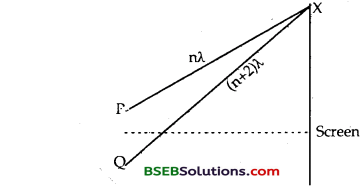Answer: Second dark (Hint: Δ = path difference = (n + 2)λ – nλ = 2λ)

Question 11. In Young’s Double Slit Experiment, the slits are 1.5 mm apart. When the slits are illuminated by a monochromatic source and the screen is kept lm apart from the slits, width of 10 fringes is measured as 3.93 mm. Calculate the wavelength of light used. What will be the width of 10 fringes when the distance between the slits and the screen is increased by 0.5 m? The source of light used remains the same. Answer: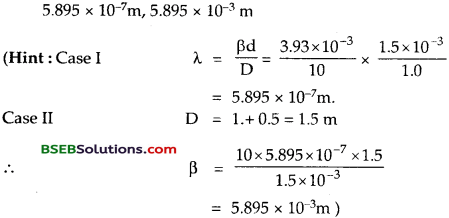Question 12. In a Young’s Double Slit experiment, the angular width of a fringe formed on distant screen is 0.1 °. The wave length of light used is 6000 A. What is the spacing between the slits? Answer: 3.44 x 10-4 mQuestion 13. In a Young’s Double Slit experiment using monochromatic light, the fringe pattern shifts by a certain distance on the screen when a mica sheet of R.Z. 1.6 and thickness 1.964 nm is introduced in the path of one of the interferring waves. The mica sheet is then removed and the distance between the plane of slits and the screen is doubled. It is found that the distance between successive maxima or minima is the same as the observed fringe shift upon the introduction of the mica sheet. Calculate the wave length of the light. Answer: λ = 5892 AQuestion 14. A slit 4.0 cm wide is irradiated with microwaves of wavelength 2.0 cm. Find the angular spread of central maximum, assuming incidence normal to the plane of the slit. Answer: 60°Question 15. Light of wavelength 5000A is incident on a double slit. If the overall separation of 10 fringes and screen 200 cm away is 1.0 cm, find the distance between the two slits. Answer: 1 mm.

Question 16. The light of wavelength 600 nm is incident normally on a slit of width 3 mm. Calculate the linear width of central maximum on a screen kept 3m away from the slit. Answer: 1.2 mm

Question 17. A slit 4.0 cm wide is irradiated with microwaves of wavelength 2.0 cm. Find the angular spread of central maxima assuming incidence normal to the plane of the slit. Answer: 60°

## Bihar Board Class 12th Physics Wave Optics Textbooks for Exam Preparations

Bihar Board Class 12th Physics Wave Optics Textbook Solutions can be of great help in your Bihar Board Class 12th Physics Wave Optics exam preparation. The BSEB STD 12th Physics Wave Optics Textbooks study material, used with the English medium textbooks, can help you complete the entire Class 12th Physics Wave Optics Books State Board syllabus with maximum efficiency.

## FAQs Regarding Bihar Board Class 12th Physics Wave Optics Textbook Solutions

#### Can we get a Bihar Board Book PDF for all Classes?

Yes you can get Bihar Board Text Book PDF for all classes using the links provided in the above article.

## Important Terms

Bihar Board Class 12th Physics Wave Optics, BSEB Class 12th Physics Wave Optics Textbooks, Bihar Board Class 12th Physics Wave Optics, Bihar Board Class 12th Physics Wave Optics Textbook solutions, BSEB Class 12th Physics Wave Optics Textbooks Solutions, Bihar Board STD 12th Physics Wave Optics, BSEB STD 12th Physics Wave Optics Textbooks, Bihar Board STD 12th Physics Wave Optics, Bihar Board STD 12th Physics Wave Optics Textbook solutions, BSEB STD 12th Physics Wave Optics Textbooks Solutions,
Share: